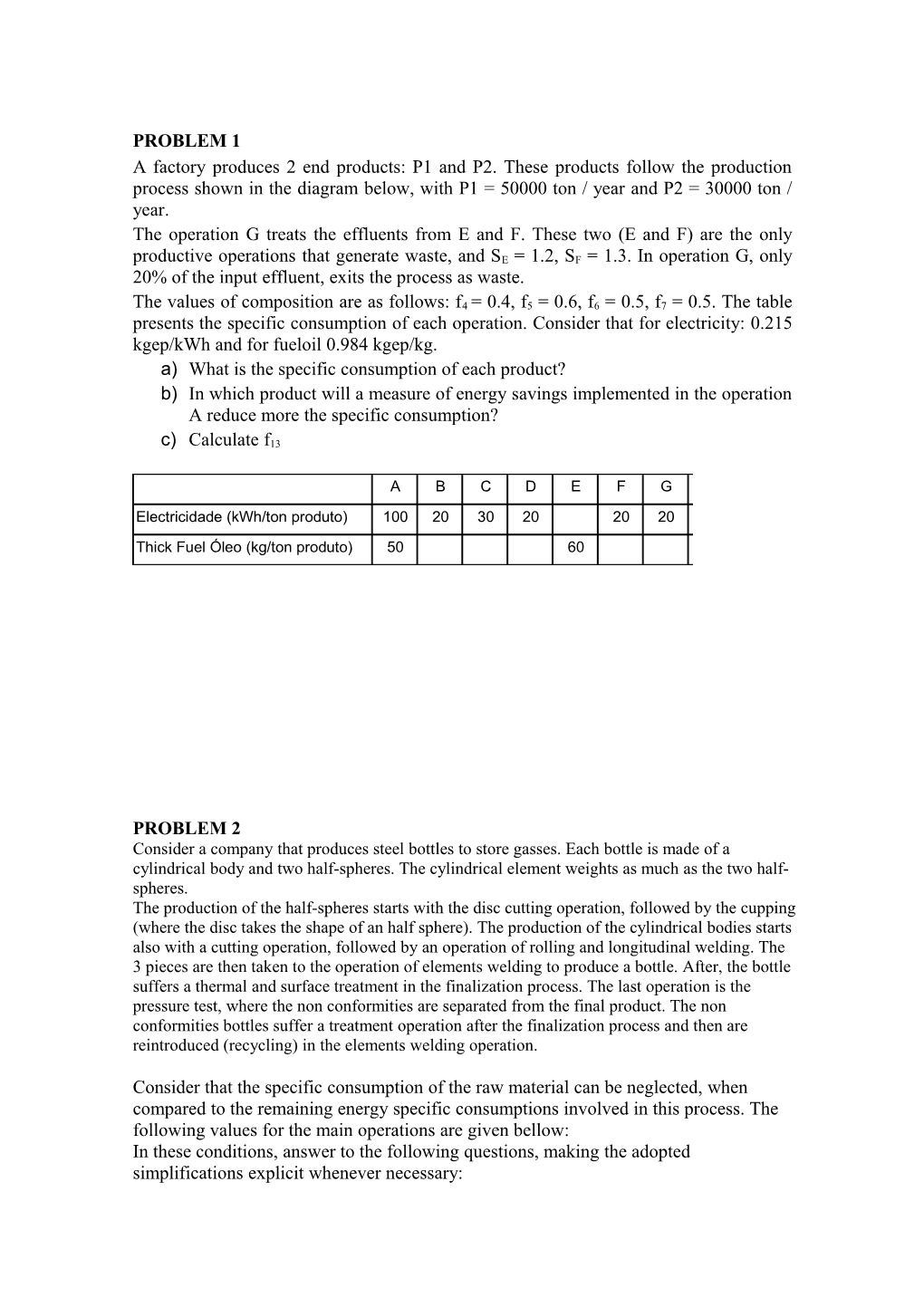# A)What Is the Specific Consumption of Each Product?##### PROBLEM 1

A factory produces 2 end products: P1 and P2. These products follow the production process shown in the diagram below, with P1 = 50000 ton / year and P2 = 30000 ton / year.

The operation G treats the effluents from E and F. These two (E and F) are the only productive operations that generate waste, and SE = 1.2, SF = 1.3. In operation G, only 20% of the input effluent, exits the process as waste.

The values of composition are as follows: f4 = 0.4, f5 = 0.6, f6 = 0.5, f7 = 0.5. The table presents the specific consumption of each operation. Consider that for electricity: 0.215 kgep/kWh and for fueloil 0.984 kgep/kg.

a)What is the specific consumption of each product?

b)In which product will a measure of energy savings implemented in the operation A reduce more the specific consumption?

c)Calculate f13

PROBLEM 2

Consider a company that produces steel bottles to store gasses. Each bottle is made of a cylindrical body and two half-spheres. The cylindrical element weights as much as the two half-spheres.

The production of the half-spheres starts with the disc cutting operation, followed by the cupping (where the disc takes the shape of an half sphere). The production of the cylindrical bodies starts also with a cutting operation, followed by an operation of rolling and longitudinal welding. The 3 pieces are then taken to the operation of elements welding to produce a bottle. After, the bottle suffers a thermal and surface treatment in the finalization process. The last operation is the pressure test, where the non conformities are separated from the final product. The non conformities bottles suffer a treatment operation after the finalization process and then are reintroduced (recycling) in the elements welding operation.

Consider that the specific consumption of the raw material can be neglected, when compared to the remaining energy specific consumptions involved in this process. The following values for the main operations are given bellow:

In these conditions, answer to the following questions, making the adopted simplifications explicit whenever necessary:

a)Draw the block diagram of the above production process.

b)Determine the expression to calculate the specific energy consumption of the bottles.

c)Determine the primary energy specific consumption associated to the bottles.

d)Indicate if the cycling operation is reasonable in terms of energy consumption, justify your answer.

e)Determine the limit value for the specific energy consumption of the recycling operation (the value that rules out the advantage of recycling in terms of energy consumption).